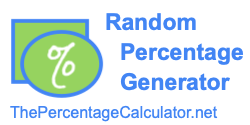Percentage RandomizerUse the Percentage Randomizer to generate a random percentage. Press "Randomize" below to get a random percentage.

The Percentage Randomizer's random percentages range from 0 to 100 percent.

The default setting is zero decimals, but you can add decimals by adding however many decimals you want in the "Decimals" box.

A new random percentage will appear every time your press the "Randomize" button.

Decimals

Random Percentage

Percentage Calculator
Go here if you want to check out the popular and best free online Percentage Calculator.